# PSAT Math : How to evaluate a fraction

## Example Questions

← Previous 1 2 Next →

### Example Question #11 : How To Evaluate A Fraction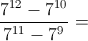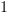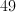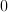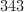Explanation:

Factor out 7 from the numerator: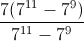This simplifies to 7.

### Example Question #11 : How To Evaluate A Fraction

Ifpizzas costdollars andsodas cost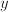dollars, what is the cost ofpizzas andsodas in terms ofand?Explanation:

If 10 pizzas cost x dollars, then each pizza costs x/10. Similarly, each soda costs y/6. We can add the pizzas and sodas together by finding a common denominator: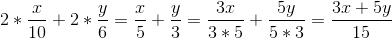### Example Question #11 : How To Evaluate A Fraction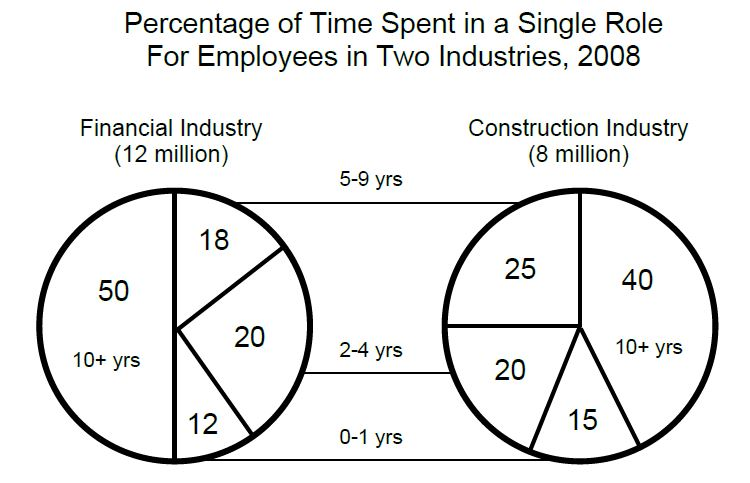According the pie chart, the degree measure of the sector representing the number of workers spending 5 to 9 years in the same role is how much greater in the construction industry chart than in the financial industry chart?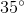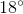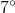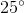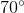Explanation:

Since the values in the pie charts are currently in terms of percentages (/100), we must convert them to degrees (/360, since within a circle) to solve the question. The "5 to 9 years" portion for the financial and construction industries are 18 and 25 percent, respectively. As such, we can cross-multiply both:

18/100 = x/360

x = 65 degrees

25/100 = y/360

y = 90 degrees

Subtract: 90 – 65 = 25 degrees

Alternatively, we could first subtract the percentages (25 – 18 = 7), then convert the 7% to degree form via the same method of cross-multiplication.

### Example Question #11 : How To Evaluate A Fraction

6 contestants have an equal chance of winning a game.  One contestant is disqualified, so now the 5 remaining contestants again have an equal chance of winning.  How much more likely is a contestant to win after the disqualification?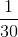Explanation:

When there are 6 people playing, each contestant has a 1/6 chance of winning.  After the disqualification, the remaining contestants have a 1/5 chance of winning.

1/5 – 1/6 = 6/30 – 5/30 = 1/30.

### Example Question #11 : How To Evaluate A Fraction

Simplify: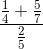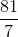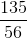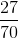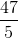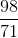Explanation:

Begin by simplifying the numerator.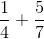has a common denominator of.  Therefore, we can rewrite it as: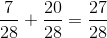Now, in our original problem this is really is: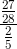When you divide by a fraction, you really multiply by the reciprocal: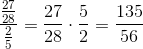### Example Question #261 : Gre Quantitative Reasoning

Simplify: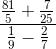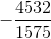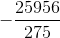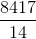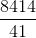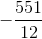Explanation:

Begin by simplifying the numerator and the denominator.

Numerator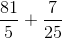has a common denominator of.  Therefore, we have: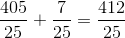Denominator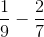has a common denominator of.  Therefore, we have: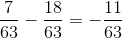Now, reconstructing our fraction, we have: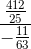To make this division work, you multiply the numerator by the reciprocal of the denominator:### Example Question #261 : Algebra

Simplify: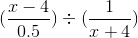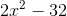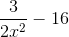None of the other answer choices are correct.Explanation:

Recall that dividing is equivalent multiplying by the reciprocal.  Therefore, ((x - 4) / (1 / 2)) / (1 / (x + 4)) = ((x - 4) * 2)  *  (x + 4) / 1.

Let's simplify this further:

(2x – 8) * (x + 4) = 2x2 – 8x + 8x – 32 = 2x2 – 32

### Example Question #11 : How To Evaluate A Fraction

Solve for: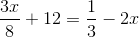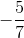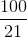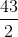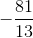Explanation:

Begin by isolating the variables: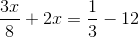Now, the common denominator of the variable terms is. The common denominator of the constant values is. Thus, you can rewrite your equation: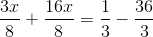Simplify: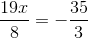Cross-multiply: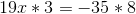Simplify: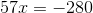Finally, solve for: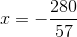← Previous 1 2 Next →

### All PSAT Math Resources